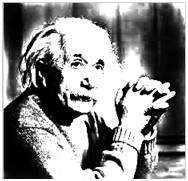# Gray Level Transformation

We have discussed some of the basic transformations in our tutorial of Basic transformation. In this tutorial we will look at some of the basic gray level transformations.

## Image enhancement

Enhancing an image provides better contrast and a more detailed image as compare to non enhanced image. Image enhancement has very applications. It is used to enhance medical images , images captured in remote sensing , images from satellite e.t.c

The transformation function has been given below

s = T ( r )

where r is the pixels of the input image and s is the pixels of the output image. T is a transformation function that maps each value of r to each value of s. Image enhancement can be done through gray level transformations which are discussed below.

## Gray level transformation

There are three basic gray level transformation.

• Linear

• Logarithmic

• Power – law

The overall graph of these transitions has been shown below.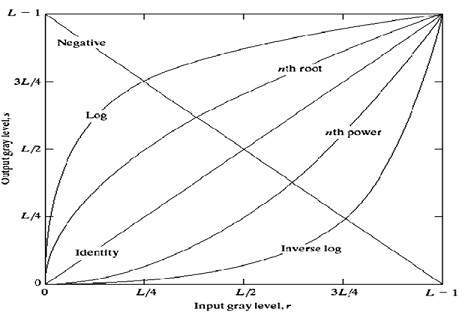## Linear transformation

First we will look at the linear transformation. Linear transformation includes simple identity and negative transformation. Identity transformation has been discussed in our tutorial of image transformation, but a brief description of this transformation has been given here.

Identity transition is shown by a straight line. In this transition, each value of the input image is directly mapped to each other value of output image. That results in the same input image and output image. And hence is called identity transformation. It has been shown below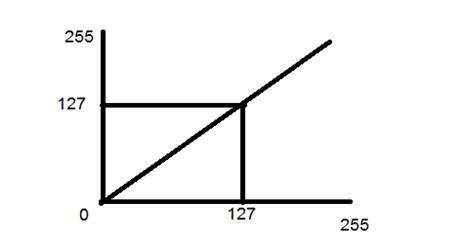## Negative transformation

The second linear transformation is negative transformation, which is invert of identity transformation. In negative transformation, each value of the input image is subtracted from the L-1 and mapped onto the output image.

The result is somewhat like this.

### Input Image### Output Image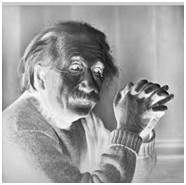In this case the following transition has been done.

s = (L – 1) – r

since the input image of Einstein is an 8 bpp image , so the number of levels in this image are 256. Putting 256 in the equation, we get this

s = 255 – r

So each value is subtracted by 255 and the result image has been shown above. So what happens is that , the lighter pixels become dark and the darker picture becomes light. And it results in image negative.

It has been shown in the graph below.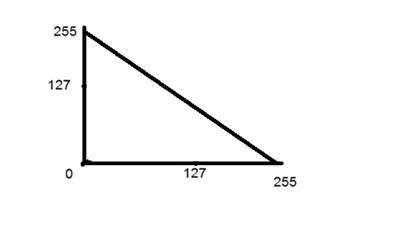## Logarithmic transformations:

Logarithmic transformation further contains two type of transformation. Log transformation and inverse log transformation.

## Log transformation

The log transformations can be defined by this formula

s = c log(r + 1).

Where s and r are the pixel values of the output and the input image and c is a constant. The value 1 is added to each of the pixel value of the input image because if there is a pixel intensity of 0 in the image, then log (0) is equal to infinity. So 1 is added , to make the minimum value at least 1.

During log transformation , the dark pixels in an image are expanded as compare to the higher pixel values. The higher pixel values are kind of compressed in log transformation. This result in following image enhancement.

The value of c in the log transform adjust the kind of enhancement you are looking for.

### Input Image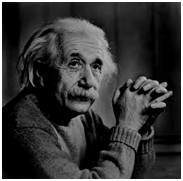### Log Tranform ImageThe inverse log transform is opposite to log transform.

## Power – Law transformations

There are further two transformation is power law transformations, that include nth power and nth root transformation. These transformations can be given by the expression:

s=cr^γ

This symbol γ is called gamma, due to which this transformation is also known as gamma transformation.

Variation in the value of γ varies the enhancement of the images. Different display devices / monitors have their own gamma correction, that’s why they display their image at different intensity.

This type of transformation is used for enhancing images for different type of display devices. The gamma of different display devices is different. For example Gamma of CRT lies in between of 1.8 to 2.5 , that means the image displayed on CRT is dark.

### Correcting gamma.

s=cr^γ

s=cr^(1/2.5)

The same image but with different gamma values has been shown here.

### For example:

Gamma = 10Gamma = 8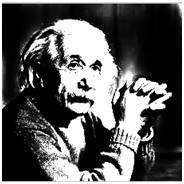Gamma = 6